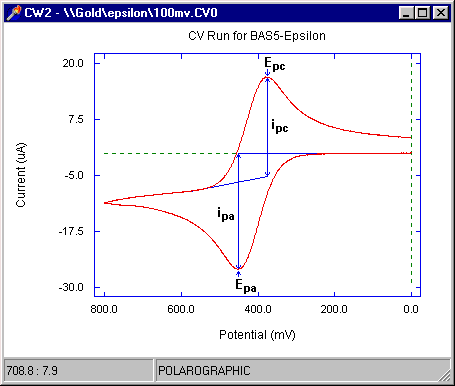# Cyclic Voltammetry - Data Analysis

The important parameters for a cyclic voltammogram are the peak potentials Ep and peak currents ip (Fig1), which are measured using the Peak Parameters operation.Figure 1. A typical cyclic voltammogram showing the important peak parameters.

If a redox system remains in equilibrium throughout the potential scan, the redox process is said to be reversible (equilibrium requires that the surface concentrations of O and R are maintained at the values required by the Nernst equation). The following parameter values are used to characterize the cyclic voltammogram of a reversible process:

1. the peak potential separation DEp (= Epc - Epa) = 59.2/n mV at all scan rates at 25 oC.
2. the peak current ratio = ipa/ipc = 1 at all scan rates
3. the peak current function ip/n1/2 (n = scan rate) is independent of n (see equation for peak current)

The peak current is given by the equation:

ip = 2.69x105n3/2ACD1/2n1/2

 where: n = number of electrons transferred/molecule A = electrode surface area (cm2) C = concentration (mol cm-3) D = diffusion coefficient (cm2 s-1)

For a reversible process, Eo' is given by the mean of the peak potentials.

Departures from reversible behavior for a redox process are shown by variations of the above parameters from the values observed for reversible processes. There are two major causes for irreversible behavior and these are discussed below.

#### 1) Slow Electron Transfer Kinetics

Reversibility requires that the electron transfer kinetics are fast enough to maintain the surface concentrations of O and R at the values required by the Nernst equation. Hence, reversibility depends on the relative values of the standard heterogeneous electron transfer rate constant (ks) and the rate of change of potential - the scan rate n. If the ratio of ks/n is sufficiently small that Nernstian concentrations cannot be maintained, then the process is said to be quasi-reversible. A quasi-reversible process is characterized by DEp > 59.2/n mV, with the value increasing with increasing n.

Since reversibility depends on the value of ks/n, it may be possible to change a process from quasi-reversible to reversible by decreasing n (which allows more time for the surface concentrations to adjust to the new values required by the changing potential). In addition, DEp depends on the value of ks/n, and ks can therefore be calculated from the variation of DEp with n.

Unfortunately, increases in DEp with increasing n can also be due to uncompensated solution resistance Ru. The effect of Ru can be minimized by careful experimental design, electronic positive feedback compensation and post-run data manipulation. The two effects can be distinguished by varying the analyte concentration (the potential drop due to uncompensated solution resistance, and hence the resulting DEp, increase with increasing current, whereas ks is independent of analyte concentration).

#### 2) Chemical Reactions of O and R

Equilibrium values of O and R can only be maintained during a cyclic voltammetry experiment if both O and R are stable on the experimental time scale. For example, if the reduction of O to R is followed by the conversion of R to P, then more R must be generated to compensate for the loss of R. Therefore, the rate of reduction increases and Epc moves to a more positive value. In addition, ipa/ipc is less than unity (since only a fraction of the molecules that were reduced on the forward scan are available for reoxidation on the reverse scan). The value of the current function can also be affected by chemical reactions following electron transfer.

The effect of a chemical reaction depends on the value of the ratio k/n (where k is the rate of the chemical reaction). If this value is large, then the chemical reaction has a significant effect, whereas any effect is much less if this ratio is small. Therefore, it may be possible to eliminate the effect of the chemical reaction (thereby restoring reversiblity) by increasing n. k can be calculated either by simulation studies or by investigating the effect of n on ipa/ipc.

Although cyclic voltammetry is very widely used for the initial redox characterization of a molecule (i.e., the redox potentials, and the stability of the different oxidations states) and for qualitative investigation of chemical reactions that accompany electron transfer, there are a number of disadvantages inherent in this technique:

1. The effects of slow heterogeneous electron transfer and chemical reactions cannot be separated. If both of these effects are present, then the rate constants for these processes can only be calculated using simulation methods.
2. There is a background charging current throughout the experiment of magnitude nCdl (where Cdl is the capacitance of the interface at the working electrode). This restricts the detection limit to about 10-5 M. In addition, the ratio of the peak faradaic current to the charging current decreases with increasing n (since ip is proportional to n1/2), and this places an upper limit on the value of n that can be used

In spite of these limitations, cyclic voltammetry is very well suited for a wide range of applications. Indeed, in some areas of research, cyclic voltammetry is one of the standard techniques used for characterization.